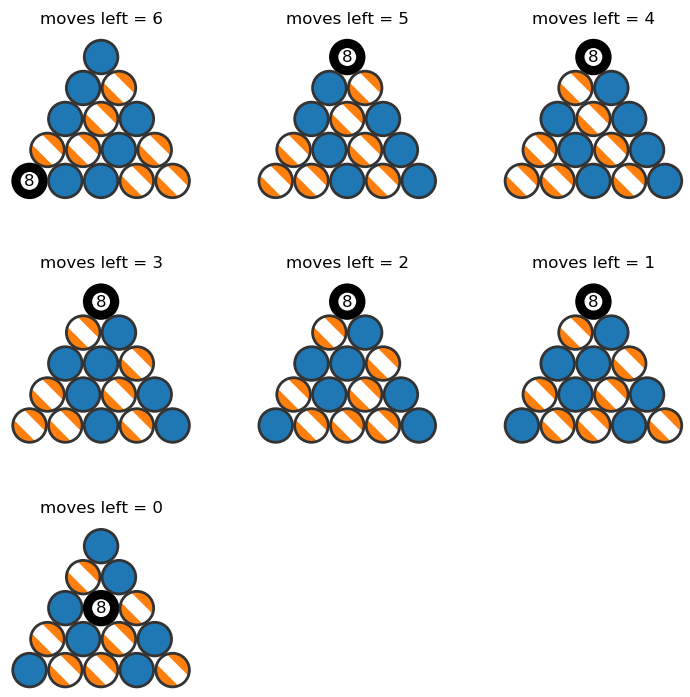# Pool hall robotsThis Riddler puzzle is about arranging pool balls using a robot!

You own a start-up, RoboRackers™, that makes robots that can rack pool balls. To operate the robot, you give it a template, such as the one shown below. (The template only recognizes the differences among stripes, solids and the eight ball. None of the other numbers matters.)First, the robot randomly corrals all of the balls into the wooden triangle. From there, the robot can either swap the location of two balls or rotate the entire rack 120 degrees in either direction. The robot continues performing these operations until the balls’ formation matches the template, and it always uses the fewest number of operations possible to do so.

Using the template given above — a correct rack for a standard game of eight-ball — what is the maximum number of operations the robot would perform? What starting position would yield this? How about the average number of operations?

Extra credit: What is the maximum number of operations the robot would perform using any template? Which template and starting position would yield this?

Here is my solution:
[Show Solution]

## 6 thoughts on “Pool hall robots”

1.Richard Holmes says:

Your solution didn’t consider the 120 degree rotation you’re allowed. Does that make a difference?

1.Laurent says:

Thanks for the comment! Upon re-reading my post I noticed I used the wrong figures by accident, so those have been updated.

To answer your question: I do consider the 120-degree rotation as a possible move in my solution. You can see in my first solution (the one with length 6) that the first move is a 120-degree clockwise rotation. Rotations aren’t common, though — I don’t think that one should never need to do more than one rotation in a shortest path.

In my remark at the very end of the post, I argue that 8 moves is an upper bound (it’s always possible to get from one position to another in 8 moves or fewer) and in demonstrating this, I use an argument that doesn’t require any rotations. That means that when the shortest path between two configurations has length 8, you don’t need to use rotations. That being said, rotations can be important for shorter paths. In the original problem, if rotations aren’t used, it’s impossible to make it in 6 moves (it takes 8). I added some text at the end of my solution to clarify.

1.Richard Holmes says:

You definitely don’t need more than one rotation, because rotations commute with swaps (if a swap is regarded as operating on two particular balls, as opposed to two particular positions). So you can arrange for all rotations to occur (for instance) first, but consecutive rotations can be replaced by a single rotation.

1.Richard Holmes says:

… I think. Though rotation definitely operates on positions, not balls, so maybe this argument leaks.

1.Guy D. Moore says:

No, the statement is correct but not quite correctly formulated.
For any swap-then-rotation, there is a rotation-then-swap which leads to the identical final state. Specifically, if you rotate first, you then swap the rotated positions of the balls you would have swapped first.
That rule lets you move all rotations to the beginning. Then it’s clear that there is no need to perform two rotations, since RR=L, LL=R, and RL=LR=identity.

2.Bryan Bischof says:

You do a nice job of explaining your solution, I would have liked to see a diagram explaining your graph. Also, maybe some of the smaller rack sizes. 🙂

Great work though!